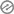# Addition of Two and Three Digit Numbers With RegroupingSubject :

Mathematics

Term :First Term

Week:

Week 8Class :

Primary 3/ Basic 3

Topic : Addition of Two and Three Digit Numbers With Regrouping

Previous lesson :

The pupils have the previous knowledge of Addition of Three Digit Numbers Without Regrouping

Behavioural objectives :

At the end of the lesson, the pupils should be able to• Add up figures that are written in three digits under Hundreds, Tens and Units
• Arrange the sums of three digit numbers correctly under Hundreds, Tens and Units without making mistakes
• Calculate the total of three digit figures without making mistakes

Instructional Materials :

• Wall charts
• Pictures
• Related Online Video
• Flash Cards
• Lagos state scheme of work on Mathematics
• Text Books
• Workbooks
• Pebbles
• Abacus

Methods of Teaching :

• Class Discussion
• Group Discussion
• Explanation
• Role Modelling
• Role DelegationReference Materials :

• Scheme of Work
• Online Information
• Textbooks
• Workbooks
• 9 Year Basic Education Curriculum
• Workbooks

Content :

1.  475 + 475 = 9502. 596 + 489 = 1085

3. 89 + 698   = 787

4. 568 + 666 = 1234

5. 586 + 695 = 1281Presentation

The topic is presented step by stepStep 1:

The class teacher revises the previous topics

Step 2.He introduces the new topic

Step 3:

The class teacher allows the pupils to give their own examples and he corrects them when the needs ariseConclusion

The class teacher wraps up or conclude the lesson by giving out short note to summarize the topic that he or she has just taught.

The class teacher also goes round to make sure that the notes are well copied or well written by the pupils.

He or she does the necessary corrections when and where  the needs arise.Evaluation

1. Increase 341 by 261
2. What is the sum of 453 and 964
3. Find the total of 253 and 268
4. Add up 897 and 222
5. 243 plus 374 is equal to _____
6. Expand 678
7. Expand 968
8. Expand 879 under Hundred Tens and Units
9. 357 = ____+50 + ___
10. 120= ____+____+____

Assignment